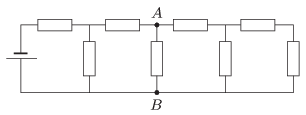Mathematical and Physical Journal
for High Schools
Issued by the MATFUND Foundation
 Already signed up? New to KöMaL?

#Problem P. 4652. (September 2014)

P. 4652. The voltage measured between points $\displaystyle A$ and $\displaystyle B$ in the circuit consisting of eight resistors, each having a resistance of $\displaystyle 100~\Omega$, is $\displaystyle 50~V$.What is the power dissipated in the system of eight resistors?

(4 pont)

Deadline expired on October 10, 2014.

### Statistics:

 109 students sent a solution. 4 points: 75 students. 3 points: 6 students. 2 points: 9 students. 1 point: 8 students. 0 point: 11 students.

Problems in Physics of KöMaL, September 2014